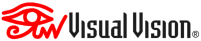Data format for RXTXeasy sendings in HTTP (manual)

RXTXeasy uses practically the same format used for TXtemp TXsoil TXdata (WiFi e GSM; click here...).
This also for MQTT.

The sending of the WiFi channel number differs (only needed if used with DisplayRX and ControlHUB) which occurs through z= instead of w=
We point out that as CGI / HTTP parameters in POST standard, the readings received by the sensors are retransmitted using the identifiers:
a0 (RXTXeasy -> readings for 433 Channell #0)
a1 (RXTXeasy -> readings for 433 Channell #1)
...
a100 (RXTXeasy -> readings for WiFi Channell #0)
a101 (RXTXeasy -> readings for WiFi Channell #1)
...
In summary, while TXtemp and TXdata send the identifier r of their single reading, RXTXeasy retransmits all the readings it collects, since there are many they do not go to r, but each to a + channel number, from a0 to a15 for RF433 channels, a100 to a199 for WiFi channels.

TXdata
Assuming to receive a123 = ..., for a channel that receives a TXdata, the data in a123 are:
- the response that the TXdata receives from its command/action (for example a response made up of bytes in HexAscii command / ModBus commands)

TXtemp
For a channel receiving a TXtemp, the data in a123 are 2 or 3 bytes in HexAscii (B1) (B2) (B3):
- temperature=((B1)*256+(B2)) / 100
- (if present) humidity=(B3)
Example for channel 0 in 433: 090A would be temperature 23.14°C or 090A15 would be temperature 23.14 ° C and humidity 21%

TXsoil
For a channel receiving a TXsoil the data in a123 are 2 or 3 bytes in HexAscii (B1) (B2) [B3]:
- (B1) = 6F (hex)
- humidity=(B2)
Or:
- temperature=((B1)*256+(B2)) / 100    (if there is no temperature measurement, the data could be between -12600 and -12800)
- humidity=(B3)
Example for channel 0 in 433: 6F15 would be humidity 21%

Here are the quick manuals for some other products:(C) 2020 VisualVision